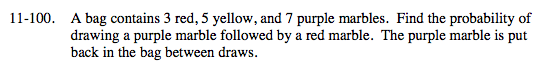### Home > MC2 > Chapter 11 > Lesson 11.3.2 > Problem11-100

11-100.What is the probability of drawing a purple marble?
What is the probability of drawing a red marble?

Multiply the two probabilities together to find the probability of both events happening.

$\frac{7}{15}\left(\frac{3}{15}\right)$

$\frac{21}{225}$

Use the eTool below to test the probability.
Click the link at right for the full version of the eTool: MC2 11-100 HW eTool